# Geospatial data

## Overview

Given a table or array containing data, we can georeference these objects onto a geospatial domain with the georef function. For a list of available domains, please see Domains.

GeoStatsBase.georefFunction
georef(table, domain)

Georeference table on geospatial domain.

georef(table, coords)

Georeference table on a PointSet(coords).

georef(table, coordnames)

Georeference table using columns coordnames.

georef(tuple, domain)

Georeference named tuple on geospatial domain.

georef(tuple, coords)

Georefrence named tuple on PointSet(coords).

georef(tuple; origin=(0.,0.,...), spacing=(1.,1.,...))

Georeference named tuple on CartesianGrid(size(tuple), origin, spacing).

In the opposite direction, the functions values and domain can be used to retrieve the table of attributes and the underlying geospatial domain.

Base.valuesFunction
values(data, [rank])

Return the values of data for a given rank as a table.

The rank is a non-negative integer that specifies the parametric dimension of the geometries of interest:

• 0 - points
• 1 - segments
• 2 - triangles, quadrangles, ...
• 3 - tetrahedrons, hexahedrons, ...

If the rank is not specified, it is assumed to be the rank of the elements of the domain.

## Examples

### Tables

Consider a table (e.g. DataFrame) with 25 samples of temperature and precipitation:

using DataFrames

table = DataFrame(T=rand(25), P=rand(25))

25 rows × 2 columns

TP
Float64Float64
10.9398590.966037
20.3102150.96439
30.1905330.251006
40.1996450.468665
50.6485430.705078
60.8431620.983003
70.3318940.24014
80.6096260.512249
90.7929210.9923
100.158490.681798
110.6065970.596699
120.9151040.791208
130.5423870.626546
140.610630.705119
150.3297980.263909
160.2816050.00982256
170.985660.959412
180.4002180.553004
190.2869370.553966
200.6936730.863948
210.237230.326765
220.3573950.070729
230.07429540.842851
240.599030.821368
250.1007060.0706933

We can georeference this table based on a given set of coordinates:

𝒟 = georef(table, PointSet(rand(2,25)))

plot(𝒟)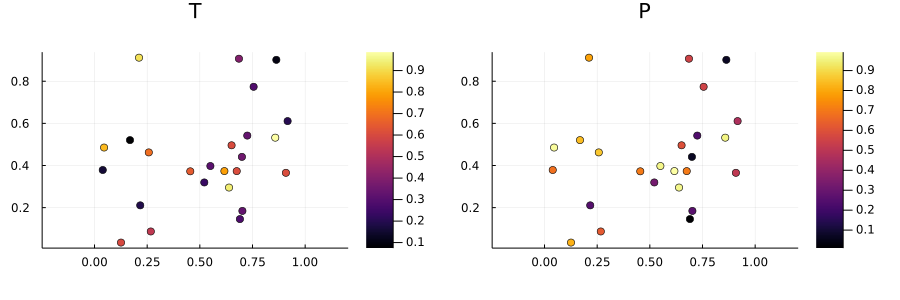or alternatively, georeference it on a 5x5 regular grid (5x5 = 25 samples):

𝒟 = georef(table, CartesianGrid(5,5))

plot(𝒟)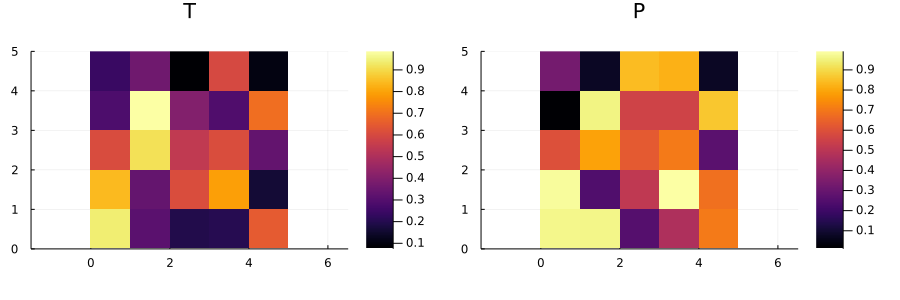In the first case, the PointSet domain type can be omitted, and GeoStats.jl will understand that the matrix passed as the second argument contains the coordinates of a point set:

𝒟 = georef(table, rand(2,25))
25 MeshData{2,Float64}
variables (rank 0)
└─P (Float64)
└─T (Float64)
domain: 25 PointSet{2,Float64}

Another common pattern in spatial data sets is when the coordinates of the samples are already part of the table as columns. In this case, we can specify the column names as symbols:

table = DataFrame(T=rand(25), P=rand(25), X=rand(25), Y=rand(25), Z=rand(25))

𝒟 = georef(table, (:X,:Y,:Z))

plot(𝒟)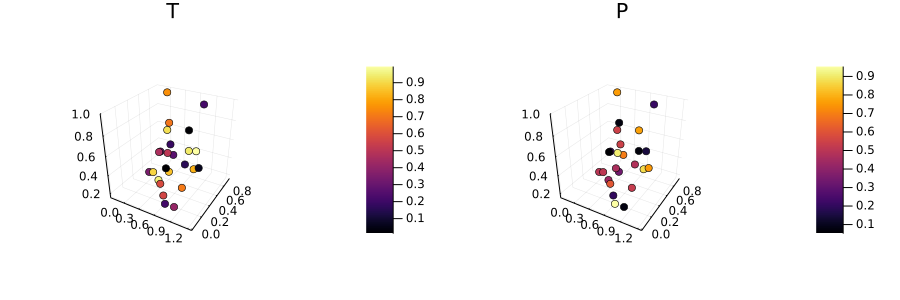### Arrays

Consider arrays (e.g. images) with data for various spatial variables. We can georeference these arrays using a named tuple, and GeoStats.jl will understand that the shape of the arrays should be preserved in a Cartesian grid:

T, P = rand(5,5), rand(5,5)

𝒟 = georef((T=T, P=P))

plot(𝒟)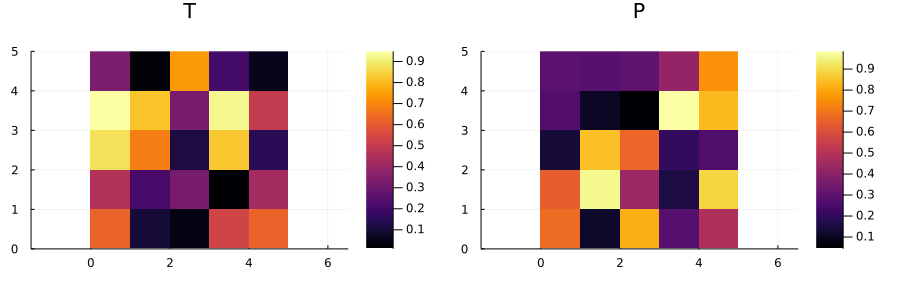We can also specify the origin and spacing of the grid using keyword arguments:

𝒟₁ = georef((T=T, P=P), origin=(0.,0.), spacing=(1.,1.))
𝒟₂ = georef((T=T, P=P), origin=(10.,10.), spacing=(2.,2.))

plot(𝒟₁)
plot!(𝒟₂)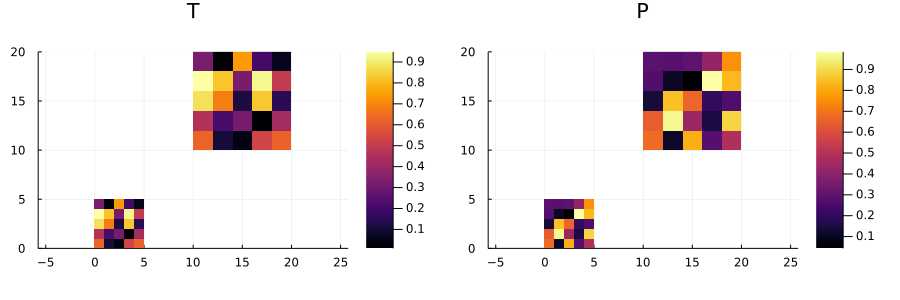Alternatively, we can interpret the entries of the named tuple as columns in a table:

𝒟 = georef((T=T, P=T), rand(2,25))

plot(𝒟)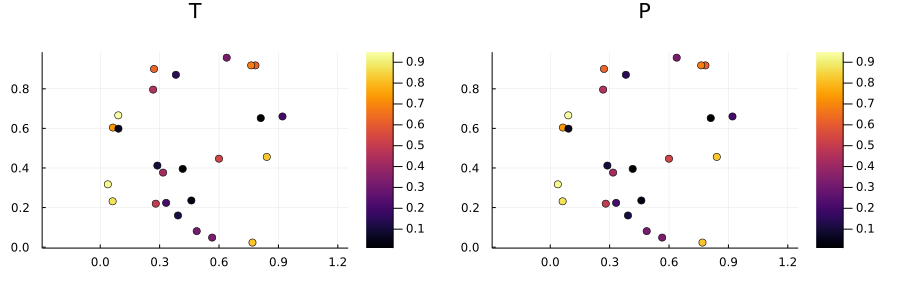### Shapefiles

The GeoTables.jl package can be used to load geospatial data from various file formats. It also provides utility functions to automatically download maps given the name of any region in the world.

We can load a shapefile as a geospatial table that is compatible with the framework:

using GeoTables

zone = GeoTables.load("data/zone.shp")
4 GeoTable{2,Float64}
variables (rank 2)
└─ACRES (Float64)
└─Hectares (Float64)
└─MACROZONA (String)
└─PERIMETER (Float64)
└─area_m2 (Float64)
domain: 4 GeometrySet{2,Float64}
path = GeoTables.load("data/path.shp")
6 GeoTable{2,Float64}
variables (rank 2)
└─ZONA (String)
domain: 6 GeometrySet{2,Float64}

Unlike in previous examples where each row of the table was associated with simple geometries (e.g. Point or Quadrangle), here we have more complicated geometries to consider:

zone.geometry
4-element Vector{Multi{2, Float64, PolyArea{2, Float64, Chain{2, Float64, Vector{Point2}}}}}:
21 Multi-PolyArea{2,Float64}
1 Multi-PolyArea{2,Float64}
1 Multi-PolyArea{2,Float64}
2 Multi-PolyArea{2,Float64}

We can visualize these geometries as the domain of the geospatial data as usual:

plot(domain(zone), fill=true, color=:gray)
plot!(domain(path), fill=true, color=:gray90)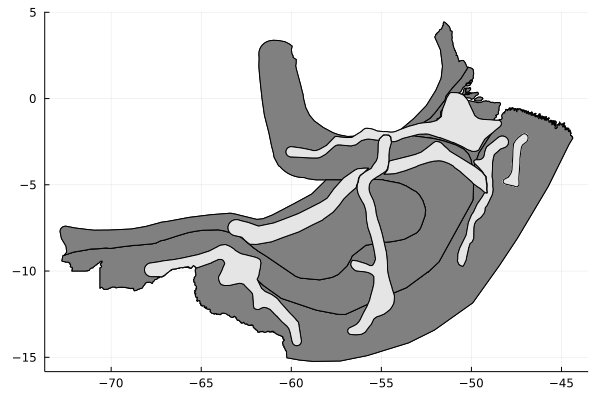and most importantly, nothing special needs to be done. This geospatial table can be used with any geostatistical workflow.

## Custom data

GeoStats.jl is integrated with the Meshes.jl project. In summary, any type that is a subtype of Meshes.Data and that implements Meshes.domain and Meshes.values is compatible with the framework and can be used in geostatistical workflows.

Please ask in our community channels if you need help implementing custom geospatial data types.